what you'll learn...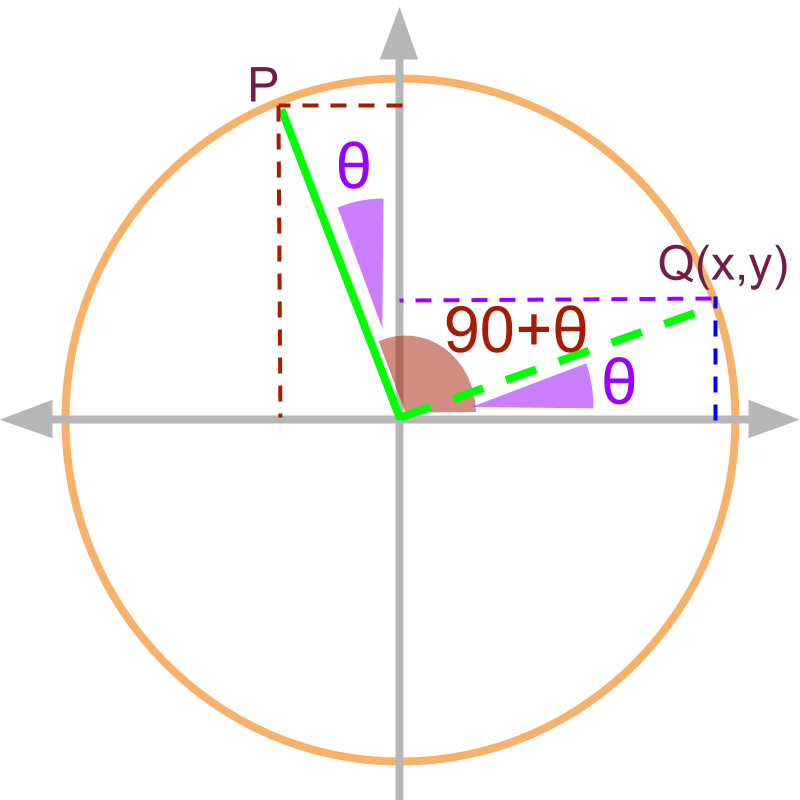»  $90+\theta$$90 + \theta$
→  $\theta$$\theta$ measured with y axis
→  complementary functions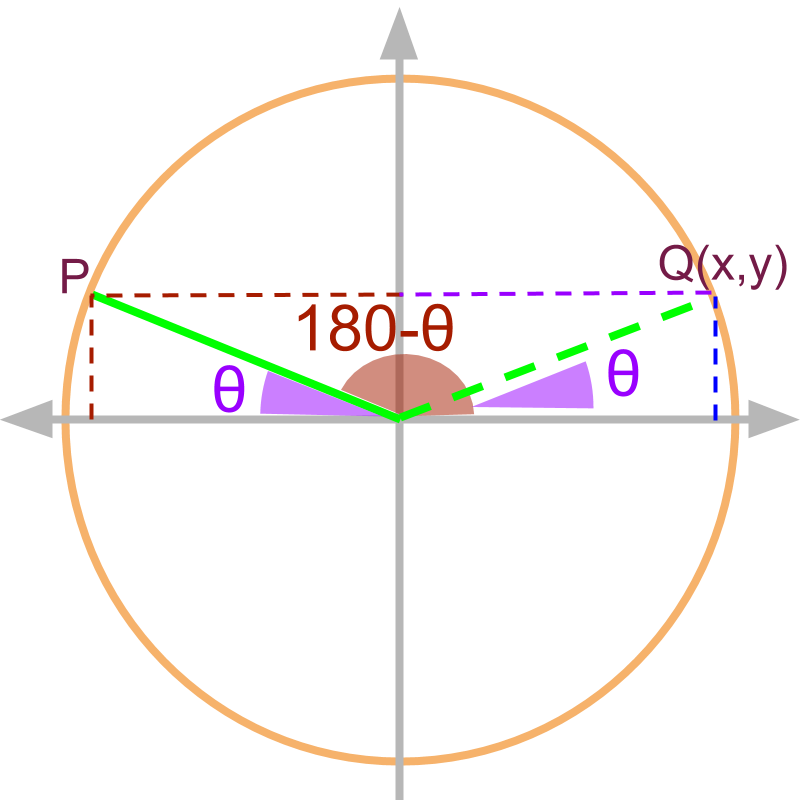»  $180-\theta$$180 - \theta$
→  $\theta$$\theta$ measured with x axis
→  equivalent functions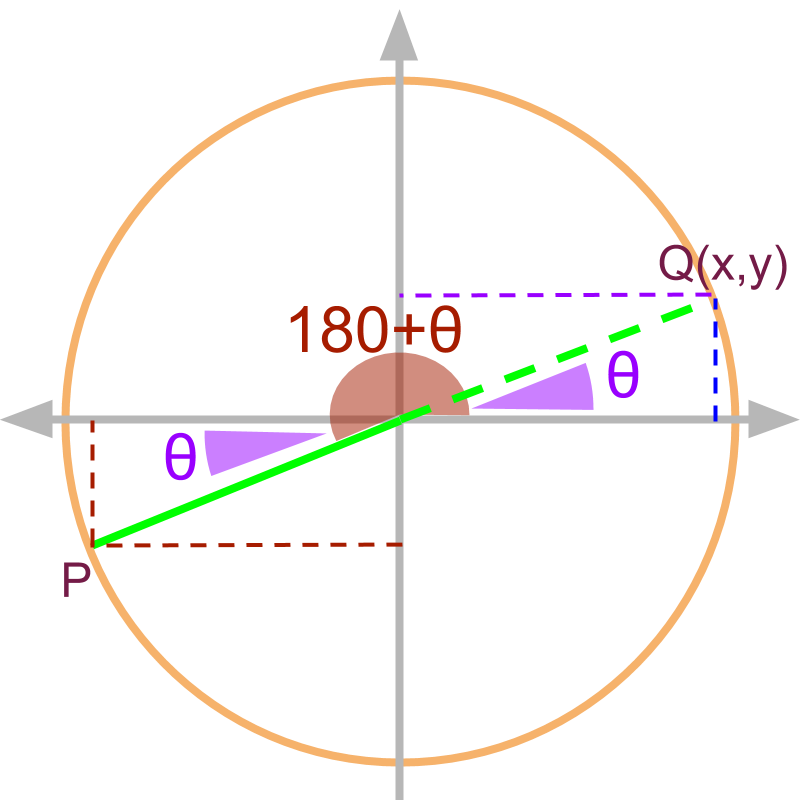»  $180+\theta$$180 + \theta$
→  $\theta$$\theta$ measured with x axis
→  equivalent functions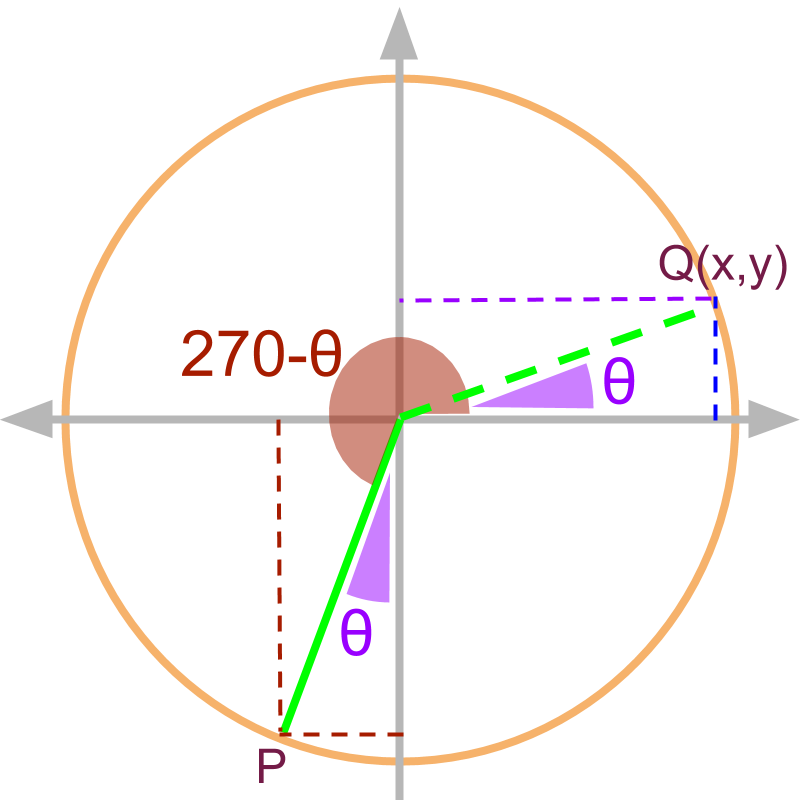»  $270-\theta$$270 - \theta$
→  $\theta$$\theta$ measured with y axis
→  complementary functions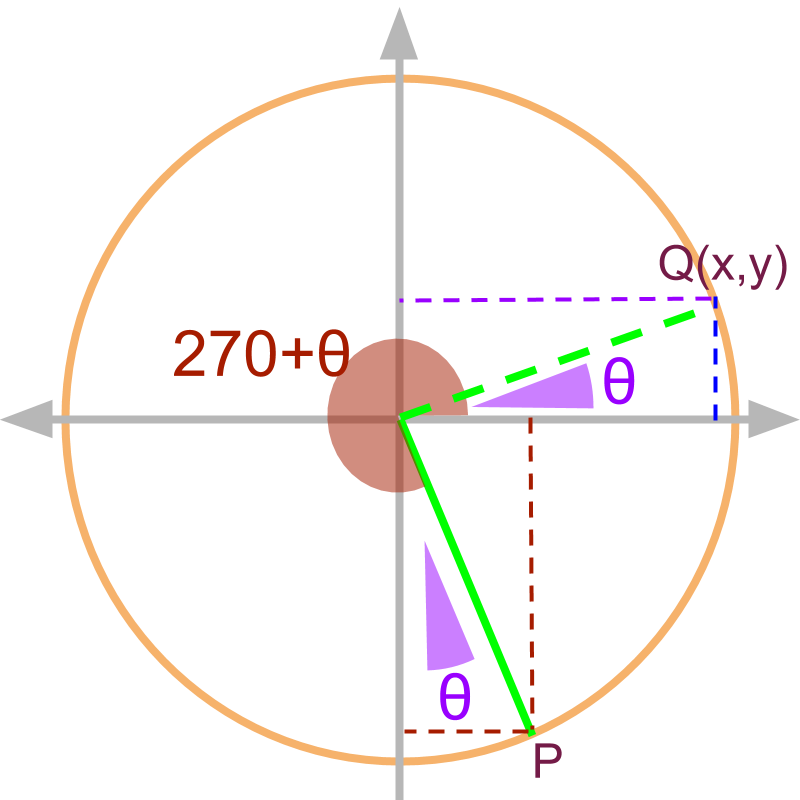»  $270+\theta$$270 + \theta$
→  $\theta$$\theta$ measured with y axis
→  complementary functions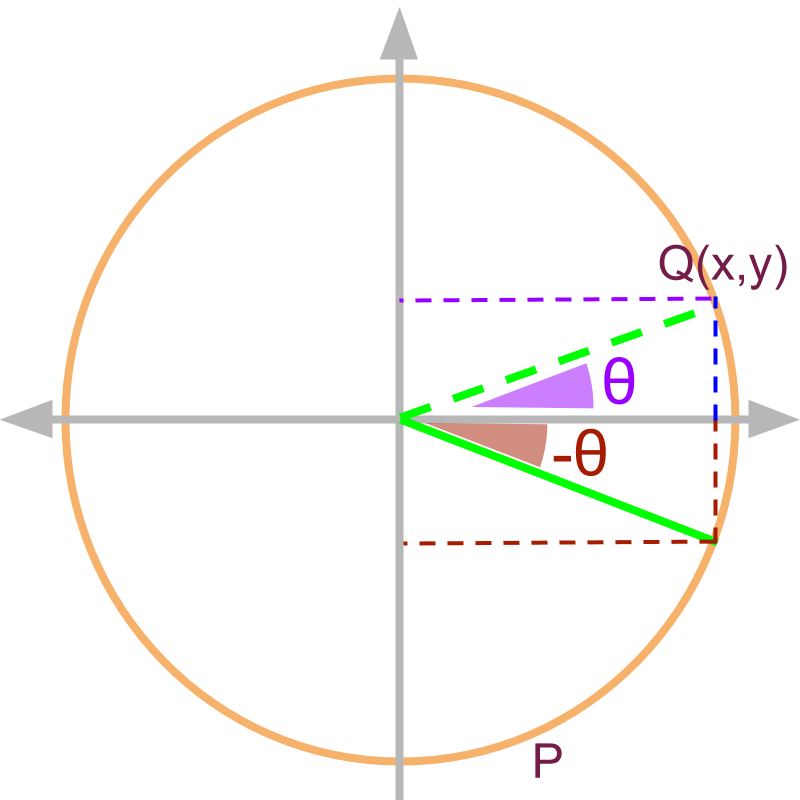»  $-\theta$$- \theta$
→  $\theta$$\theta$ measured with x axis
→  equivalent functions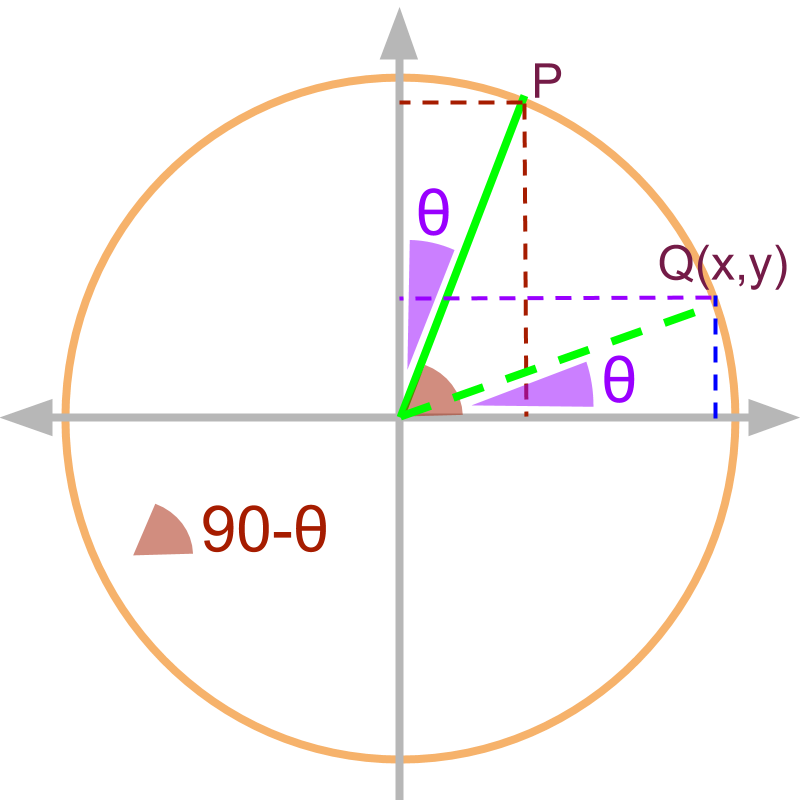»  $90-\theta$$90 - \theta$
→  $\theta$$\theta$ measured with y axis
→  complementary functionsThe angle $\omega =90+\theta$$\omega = 90 + \theta$ is shown in the figure as point P. The similar triangle with angle theta is given by $Q\left(x,y\right)$$Q \left(x , y\right)$ in 1st quadrant. Note: Given $Q\left(x,y\right)$$Q \left(x , y\right)$, the coordinate of $P$$P$ is $\left({P}_{x},{P}_{y}\right)$$\left(\textcolor{c \mathmr{and} a l}{{P}_{x}} , \textcolor{\mathrm{de} e p s k y b l u e}{{P}_{y}}\right)$:
${P}_{x}=-y$$\textcolor{c \mathmr{and} a l}{{P}_{x} = - y}$ and ${P}_{y}=x$$\textcolor{\mathrm{de} e p s k y b l u e}{{P}_{y} = x}$

$\mathrm{tan}\left(90+\theta \right)=\frac{-x}{y}=-\mathrm{cot}\theta$$\tan \left(90 + \theta\right) = \frac{- x}{y} = - \cot \theta$

$\mathrm{tan}\left(90+\theta \right)$$\tan \left(90 + \theta\right)$ (tan of the given angle)
$\quad = \frac{{P}_{y}}{{P}_{x}}$ (by definition of $\mathrm{tan}$$\tan$)
$\quad = \frac{x}{- y}$ (substituting the values)
$\quad = - \cot \theta$ (equivalently in 1st quadrant.)

Note: learners can work out this for $\mathrm{sin}$$\sin$ and $\mathrm{cos}$$\cos$.

For angles given as $90+\theta$$90 + \theta$,

•  the angle is in 2nd quadrant, so x projection is negative and y projection is positive.

•  the $\angle \theta$$\angle \theta$ is measured with y-axis, and so the similar triangle in 1st quadrant will have $\angle \theta$$\angle \theta$ with x-axis, thereby swapping the x and y projections.

the trigonometric ratios are:

• $\mathrm{sin}\left(90+\theta \right)=\mathrm{cos}\theta$$\sin \left(90 + \theta\right) = \cos \theta$

• $\mathrm{cos}\left(90+\theta \right)=-\mathrm{sin}\theta$$\cos \left(90 + \theta\right) = - \sin \theta$

• $\mathrm{tan}\left(90+\theta \right)=-\mathrm{cot}\theta$$\tan \left(90 + \theta\right) = - \cot \theta$The angle $\omega =180-\theta$$\omega = 180 - \theta$ is shown in the figure as point P. The similar triangle with $\angle \theta$$\angle \theta$ is given by $Q\left(x,y\right)$$Q \left(x , y\right)$ in 1st quadrant.

Note: Given $Q\left(x,y\right)$$Q \left(x , y\right)$, the coordinates of $P$$P$ is $\left({P}_{x},{P}_{y}\right)$$\left(\textcolor{c \mathmr{and} a l}{{P}_{x}} , \textcolor{\mathrm{de} e p s k y b l u e}{{P}_{y}}\right)$:
${P}_{x}=-x$$\textcolor{c \mathmr{and} a l}{{P}_{x} = - x}$ and ${P}_{y}=y$$\textcolor{\mathrm{de} e p s k y b l u e}{{P}_{y} = y}$

$\mathrm{tan}\left(180-\theta \right)=\frac{y}{-x}=-\mathrm{tan}\theta$$\tan \left(180 - \theta\right) = \frac{y}{- x} = - \tan \theta$

$\mathrm{tan}\left(180-\theta \right)$$\tan \left(180 - \theta\right)$ (tan of the given angle)
$\quad = \frac{{P}_{y}}{{P}_{x}}$ (by definition of $\mathrm{tan}$$\tan$)
$\quad = \frac{y}{- x}$ (substituting the values)
$\quad = - \tan \theta$ (equivalently in 1st quadrant.)

Note: learners can work out this for $\mathrm{sin}$$\sin$ and $\mathrm{cos}$$\cos$.

summary

For angles given as $180-\theta$$180 - \theta$, the trigonometric ratios are:

• $\mathrm{sin}\left(180-\theta \right)=\mathrm{sin}\theta$$\sin \left(180 - \theta\right) = \sin \theta$

• $\mathrm{cos}\left(180-\theta \right)=-\mathrm{cos}\theta$$\cos \left(180 - \theta\right) = - \cos \theta$

• $\mathrm{tan}\left(180-\theta \right)=-\mathrm{tan}\theta$$\tan \left(180 - \theta\right) = - \tan \theta$The angle $\omega =180+\theta$$\omega = 180 + \theta$ is shown in the figure as point P. The similar triangle with angle theta is given by $Q\left(x,y\right)$$Q \left(x , y\right)$ in 1st quadrant.

Note: Given $Q\left(x,y\right)$$Q \left(x , y\right)$, the coordinates of $P$$P$ is $\left({P}_{x},{P}_{y}\right)$$\left(\textcolor{c \mathmr{and} a l}{{P}_{x}} , \textcolor{\mathrm{de} e p s k y b l u e}{{P}_{y}}\right)$:
${P}_{x}=-x$$\textcolor{c \mathmr{and} a l}{{P}_{x} = - x}$ and ${P}_{y}=-y$$\textcolor{\mathrm{de} e p s k y b l u e}{{P}_{y} = - y}$

$\mathrm{tan}\left(180+\theta \right)=-\frac{y}{-x}=\mathrm{tan}\theta$$\tan \left(180 + \theta\right) = - \frac{y}{-} x = \tan \theta$

$\mathrm{tan}\left(180+\theta \right)$$\tan \left(180 + \theta\right)$ (tan of the given angle)
$\quad = \frac{{P}_{y}}{{P}_{x}}$ (by definition of $\mathrm{tan}$$\tan$)
$\quad = \frac{- y}{- x}$ (substituting the values)
$\quad = \tan \theta$ (equivalently in 1st quadrant.)

Note: learners can work out this for $\mathrm{sin}$$\sin$ and $\mathrm{cos}$$\cos$.

For angles given as $180+\theta$$180 + \theta$, the trigonometric ratios are:

• $\mathrm{sin}\left(180+\theta \right)=-\mathrm{sin}\theta$$\sin \left(180 + \theta\right) = - \sin \theta$

• $\mathrm{cos}\left(180+\theta \right)=-\mathrm{cos}\theta$$\cos \left(180 + \theta\right) = - \cos \theta$

• $\mathrm{tan}\left(180+\theta \right)=\mathrm{tan}\theta$$\tan \left(180 + \theta\right) = \tan \theta$The angle $\omega =270-\theta$$\omega = 270 - \theta$ is shown in the figure as point P. The similar triangle with angle theta is given by $Q\left(x,y\right)$$Q \left(x , y\right)$ in 1st quadrant.

Note: Given $Q\left(x,y\right)$$Q \left(x , y\right)$, the coordinates of $P$$P$ is $\left({P}_{x},{P}_{y}\right)$$\left(\textcolor{c \mathmr{and} a l}{{P}_{x}} , \textcolor{\mathrm{de} e p s k y b l u e}{{P}_{y}}\right)$:
${P}_{x}=-y$$\textcolor{c \mathmr{and} a l}{{P}_{x} = - y}$ and ${P}_{y}=-x$$\textcolor{\mathrm{de} e p s k y b l u e}{{P}_{y} = - x}$

What is $\mathrm{tan}\left(270-\theta \right)=\frac{-x}{-y}=\mathrm{cot}\theta$$\tan \left(270 - \theta\right) = \frac{- x}{- y} = \cot \theta$

$\mathrm{tan}\left(270-\theta \right)$$\tan \left(270 - \theta\right)$ (tan of the given angle)
$\quad = \frac{{P}_{y}}{{P}_{x}}$ (by definition of $\mathrm{tan}$$\tan$)
$\quad = \frac{- x}{- y}$ (substituting the values)
$\quad = \cot \theta$ (equivalently in 1st quadrant.)

Note: learners can work out this for $\mathrm{sin}$$\sin$ and $\mathrm{cos}$$\cos$.

summary

For angles given as $270-\theta$$270 - \theta$, the trigonometric ratios are:

• $\mathrm{sin}\left(270-\theta \right)=-\mathrm{cos}\theta$$\sin \left(270 - \theta\right) = - \cos \theta$

• $\mathrm{cos}\left(270-\theta \right)=-\mathrm{sin}\theta$$\cos \left(270 - \theta\right) = - \sin \theta$

• $\mathrm{tan}\left(270-\theta \right)=\mathrm{cot}\theta$$\tan \left(270 - \theta\right) = \cot \theta$The angle $\omega =270+\theta$$\omega = 270 + \theta$ is shown in the figure as point P. The similar triangle with angle theta is given by $Q\left(x,y\right)$$Q \left(x , y\right)$ in 1st quadrant.

Note: Given $Q\left(x,y\right)$$Q \left(x , y\right)$, the coordinates of $P$$P$ is $\left({P}_{x},{P}_{y}\right)$$\left(\textcolor{c \mathmr{and} a l}{{P}_{x}} , \textcolor{\mathrm{de} e p s k y b l u e}{{P}_{y}}\right)$:
${P}_{x}=y$$\textcolor{c \mathmr{and} a l}{{P}_{x} = y}$ and ${P}_{y}=-x$$\textcolor{\mathrm{de} e p s k y b l u e}{{P}_{y} = - x}$

$\mathrm{tan}\left(270+\theta \right)=\frac{-x}{y}=-\mathrm{cot}\theta$$\tan \left(270 + \theta\right) = \frac{- x}{y} = - \cot \theta$

$\mathrm{tan}\left(270+\theta \right)$$\tan \left(270 + \theta\right)$ (tan of the given angle)
$\quad = \frac{{P}_{y}}{{P}_{x}}$ (by definition of $\mathrm{tan}$$\tan$)
$\quad = \frac{- x}{y}$ (substituting the values)
$\quad = - \cot \theta$ (equivalently in 1st quadrant.)

Note: learners can work out this for $\mathrm{sin}$$\sin$ and $\mathrm{cos}$$\cos$.

For angles given as $270+\theta$$270 + \theta$, the trigonometric ratios are:

• $\mathrm{sin}\left(270+\theta \right)=-\mathrm{cos}\theta$$\sin \left(270 + \theta\right) = - \cos \theta$

• $\mathrm{cos}\left(270+\theta \right)=\mathrm{sin}\theta$$\cos \left(270 + \theta\right) = \sin \theta$

• $\mathrm{tan}\left(270+\theta \right)=-\mathrm{cot}\theta$$\tan \left(270 + \theta\right) = - \cot \theta$The angle $\omega =-\theta$$\omega = - \theta$ is shown in the figure as point P. The similar triangle with angle theta is given by $Q\left(x,y\right)$$Q \left(x , y\right)$ in 1st quadrant.

Note: Given $Q\left(x,y\right)$$Q \left(x , y\right)$, the coordinates of $P$$P$ is $\left({P}_{x},{P}_{y}\right)$$\left(\textcolor{c \mathmr{and} a l}{{P}_{x}} , \textcolor{\mathrm{de} e p s k y b l u e}{{P}_{y}}\right)$:
${P}_{x}=x$$\textcolor{c \mathmr{and} a l}{{P}_{x} = x}$ and ${P}_{y}=-y$$\textcolor{\mathrm{de} e p s k y b l u e}{{P}_{y} = - y}$

$\mathrm{tan}\left(-\theta \right)=\frac{-y}{x}=-\mathrm{tan}\theta$$\tan \left(- \theta\right) = \frac{- y}{x} = - \tan \theta$

$\mathrm{tan}\left(-\theta \right)$$\tan \left(- \theta\right)$ (tan of the given angle)
$\quad = \frac{{P}_{y}}{{P}_{x}}$ (by definition of $\mathrm{tan}$$\tan$)
$\quad = \frac{- y}{x}$ (substituting the values)
$\quad = - \tan \theta$ (equivalently in 1st quadrant.)

Note: learners can work out this for $\mathrm{sin}$$\sin$ and $\mathrm{cos}$$\cos$.

summary

For angles given as $-\theta$$- \theta$, the trigonometric ratios are:

• $\mathrm{sin}\left(-\theta \right)=-\mathrm{sin}\theta$$\sin \left(- \theta\right) = - \sin \theta$

• $\mathrm{cos}\left(-\theta \right)=\mathrm{cos}\theta$$\cos \left(- \theta\right) = \cos \theta$

• $\mathrm{tan}\left(-\theta \right)=-\mathrm{tan}\theta$$\tan \left(- \theta\right) = - \tan \theta$

negative angleThe angle $\omega =90-\theta$$\omega = 90 - \theta$ is shown in the figure as point P. The similar triangle with angle theta is given by $Q\left(x,y\right)$$Q \left(x , y\right)$ in 1st quadrant.

For angles given as $90-\theta$$90 - \theta$, the trigonometric ratios are:

• $\mathrm{sin}\left(90-\theta \right)=\mathrm{cos}\theta$$\sin \left(90 - \theta\right) = \cos \theta$

• $\mathrm{cos}\left(90-\theta \right)=\mathrm{sin}\theta$$\cos \left(90 - \theta\right) = \sin \theta$

• $\mathrm{tan}\left(90-\theta \right)=\mathrm{cot}\theta$$\tan \left(90 - \theta\right) = \cot \theta$

For angles measured in reference to x axis, the trigonometric ratios remain the same when equivalently represented in the 1st quadrant.

For angles measured in reference to y axis, the trigonometric ratios are equivalently complementary ratios in the 1st quadrant.

summary

For Angles in reference to x-axis:
$\mathrm{sin}\left(180+\theta \right)=-\mathrm{sin}\theta$$\sin \left(180 + \theta\right) = - \sin \theta$
$\mathrm{sin}\left(180-\theta \right)=\mathrm{sin}\theta$$\sin \left(180 - \theta\right) = \sin \theta$
$\mathrm{cos}\left(180±\theta \right)=-\mathrm{cos}\theta$$\cos \left(180 \pm \theta\right) = - \cos \theta$
$\mathrm{tan}\left(180+\theta \right)=\mathrm{tan}\theta$$\tan \left(180 + \theta\right) = \tan \theta$

$\mathrm{tan}\left(180-\theta \right)=-\mathrm{tan}\theta$$\tan \left(180 - \theta\right) = - \tan \theta$

$\mathrm{sin}\left(-\theta \right)=-\mathrm{sin}\theta$$\sin \left(- \theta\right) = - \sin \theta$
$\mathrm{cos}\left(-\theta \right)=\mathrm{cos}\theta$$\cos \left(- \theta\right) = \cos \theta$
$\mathrm{tan}\left(-\theta \right)=-\mathrm{tan}\theta$$\tan \left(- \theta\right) = - \tan \theta$

For Angles in reference to y-axis:
$\mathrm{sin}\left(90±\theta \right)=\mathrm{cos}\theta$$\sin \left(90 \pm \theta\right) = \cos \theta$
$\mathrm{cos}\left(90+\theta \right)=-\mathrm{sin}\theta$$\cos \left(90 + \theta\right) = - \sin \theta$
$\mathrm{cos}\left(90-\theta \right)=\mathrm{sin}\theta$$\cos \left(90 - \theta\right) = \sin \theta$
$\mathrm{tan}\left(90+\theta \right)=-\mathrm{cot}\theta$$\tan \left(90 + \theta\right) = - \cot \theta$

$\mathrm{tan}\left(90-\theta \right)=+\mathrm{cot}\theta$$\tan \left(90 - \theta\right) = + \cot \theta$

$\mathrm{sin}\left(270±\theta \right)=-\mathrm{cos}\theta$$\sin \left(270 \pm \theta\right) = - \cos \theta$
$\mathrm{cos}\left(270±\theta \right)=\mathrm{sin}\theta$$\cos \left(270 \pm \theta\right) = \sin \theta$
$\mathrm{tan}\left(270±\theta \right)=-\mathrm{tan}\theta$$\tan \left(270 \pm \theta\right) = - \tan \theta$

Outline

It is advised to do the firmfunda version of "basics of Trigonometry" course before doing this.

The outline of material to learn "Advanced Trigonometry" is as follows.
Note: go to detailed outline of Advanced Trigonometry

→   Unit Circle form of Trigonmetric Values

→   Trigonometric Values in all Quadrants

→   Trigonometric Values or any Angles : First Principles

→   Understanding Trigonometric Values in First Quadrant

→   Trigonometric Values in First Quadrant

→   Trigonometric Values of Compound Angles: Geometrical Proof

→   Trigonometric Values of Compound Angles: Algebraic Proof

→   Trigonometric Values of Compound Angles: tan cot

→   Trigonometric Values of Compound Angles: more results